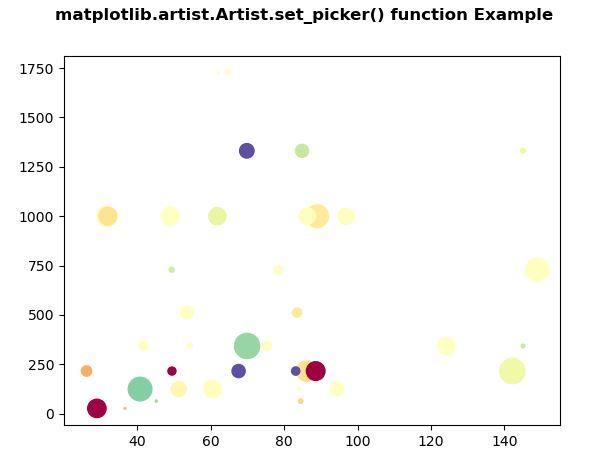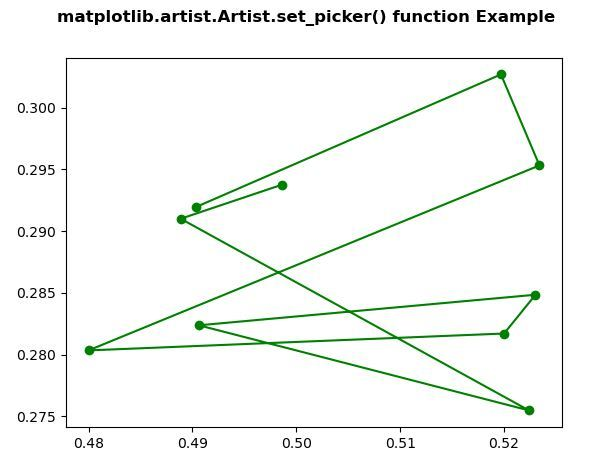# Matplotlib.artist.Artist.set_picker() in Python

• Last Updated : 10 May, 2020

Matplotlib is a library in Python and it is numerical – mathematical extension for NumPy library. The Artist class contains Abstract base class for objects that render into a FigureCanvas. All visible elements in a figure are subclasses of Artist.

## matplotlib.artist.Artist.set_picker() method

Attention geek! Strengthen your foundations with the Python Programming Foundation Course and learn the basics.

To begin with, your interview preparations Enhance your Data Structures concepts with the Python DS Course. And to begin with your Machine Learning Journey, join the Machine Learning - Basic Level Course

The set_picker() method in artist module of matplotlib library is used to define the picking behavior of the artist.

Syntax: Artist.set_picker(self, picker)

Parameters: This method accept the following parameters as discussed below:

• picker : This parameter is used to set picking behavior. This can be None or bool or float or function.

Returns: This method the picking behavior of the artist.

Below examples illustrate the matplotlib.artist.Artist.set_picker() function in matplotlib:

Example 1:

 `# Implementation of matplotlib function``from` `matplotlib.artist ``import` `Artist``import` `numpy as np ``import` `matplotlib.pyplot as plt ``      ` ` ` `np.random.seed(``19680801``) `` ` `volume ``=` `np.random.rayleigh(``27``, size ``=` `40``) ``amount ``=` `np.random.poisson(``7``, size ``=` `40``) ``ranking ``=` `np.random.normal(size ``=` `40``) ``price ``=` `np.random.uniform(``1``, ``7``, size ``=` `40``) ``      ` `fig, ax ``=` `plt.subplots() ``      ` `scatter ``=` `ax.scatter(volume ``*` `2``,  ``                     ``amount``*``*``3``, ``                     ``c ``=` `ranking``*``*``3``, ``                     ``s ``=` `price``*``*``3``, ``                     ``vmin ``=` `-``3``, ``                     ``vmax ``=` `3``, ``                     ``cmap ``=``"Spectral"``) ``   ` `Artist.set_picker(ax, picker ``=` `4``) ``         ` `fig.suptitle('matplotlib.artist.Artist.set_picker()\``function Example', fontweight ``=``"bold"``) `` ` `plt.show()`

Output:Example 2:

 `# Implementation of matplotlib function``from` `matplotlib.artist ``import` `Artist``import` `numpy as np ``import` `matplotlib.pyplot as plt `` ` `   ` `X ``=` `np.random.rand(``10``, ``200``) ``xs ``=` `np.mean(X, axis ``=` `1``) ``ys ``=` `np.std(X, axis ``=` `1``) ``   ` `fig ``=` `plt.figure() ``ax ``=` `fig.add_subplot(``111``) ``line, ``=` `ax.plot(xs, ys, ``'go-'``) ``   ` `Artist.set_picker(ax, picker ``=` `True``) ``         ` `fig.suptitle('matplotlib.artist.Artist.set_picker()\`` ``function Example', fontweight ``=``"bold"``) `` ` `plt.show()`

Output:My Personal Notes arrow_drop_up